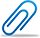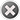Cash-back offer from 25th to 30th September 2022. Get flat 10% cash-back credited to your account for a minimum transaction of \$50. Post Your Question Today!

Question Details Normal
\$ 50.00

# STATS 200 quiz number two

• From Mathematics, Statistics
• Due on 20 Feb, 2015 09:37:00
• Asked On 16 Feb, 2015 02:39:59
• Due date has already passed, but you can still post solutions.
Question posted bySTAT 200 Quiz 2

1. (1)  The singular form of the word “dice” is “die”. Tom was throwing a six-sided die. The first time he threw, he got a three; the second time he threw, he got a three again. What’s the probability of getting a three at the third time?

2. (2)  There are 30 table tennis balls in the box: 6 are green, 10 are red, and 14 are yellow. If you shake the box and then randomly select one ball from the box, what’s the probability that you will get a red one:

3. (3) Rachel was flipping a coin with Jerry. She told jerry: “I am able to get all heads in two tosses.” Jerry laughed at her: “No, the probability of getting two heads at two tosses is only__”

4. (4)  Jennie and Alex both wanted to get a free ticket for a College Music concert. However, the concert staff told them the tickets were limited. Twenty people wanted to attend the concert but only 10 free tickets were left. So the concert center staff decided to use a lottery to decide who would receive the free tickets. What’s the probability of Jennie and Alex both getting free tickets?

5. (5) Laura and Melissa were playing dice. What the probability of Laura and Melissa both getting a 6?

6. (6)  In a statistics class with 36 students, the professor wanted to know the probability that at least two students share the same birthday. The probability will be___

a. 0.1

b. Much smaller than 0.1

c. Much bigger than 0.1

d. Not possible

7. (7)  If you throw a die for two times, what is the probability that you will get a one on the first throw or a one on the second throw (or both)?

8. (8)  Jerry got a box full of colorful candy balls. There were 50 of them: 20 red, 10 green, 12 yellow and 8 blue. After shaking the box, he randomly selected 2 candy balls from the box. What’s the probability that the first one was blue and the second one was yellow?

9. (9)  Jerry got a box full of colorful candy balls. There were 50 of them: 20 red, 10 green, 12 yellow and 8 blue. After shaking the box, he randomly selected 2 candy balls from the box. What’s the probability that the first is blue and the second one is also blue?

10.  (10) Which activity could probabilities be computed using a Binomial Distribution?

a. Flipping a coin a 100 times

b. Throwing a die one hundred times

c. The probability of getting a heart while playing card games

d. Grades earned by 100 students on a statistics final exam

11.  (11) Many researchers have argued that the TB skin test is not accurate. Imagine that the TB skin test is only 70% accurate. Sarah is thinking about having the test. Before she has the test she wonders the probability that she has TB. The probability of Sarah having TB is …

a. 70%

b. 35%

c. 30%

12.  (12) Imagine that the diabetic test accurately indicates the disease in 95% of the people who have it. What’s the miss rate?

13.  (15) Which of the following is the probability that subjects do not have the disease, but the test result is positive?

a. Miss rate

b. False positive rate

c. Base rate

d. Disease rate

14.  (16) In a normal distribution, the median is ____it’s mean and mode.

a. Approximately equal to

b. Bigger than

c. Smaller than

d. Unrelated to

15.  (17) In a normal distribution, __ percentage of the area under the curve is within one standard deviations of the mean?

a. 68%

b. 100%

c. 95%

d. It depends on the values of the mean and standard deviation

16.  (18) A normal distribution with a mean of 15 and standard deviation of 5. 95% of its area is within__

a. One standard deviation of the mean

b. Two standard deviations of the mean

c. Three standard deviations of the mean

d. It depends on the value of the mode

17.  (19)The mean of a standard normal distribution is:

a. 0

b. 1.0

c. -1.0

d. 100

18.  (20) The standard deviation of the mean for a standard distribution is:

a. 0.0

b. 1.0

c. 100

d. 68%

19.  (21) A normal distribution with a mean of 25 and standard deviation of 5. What is the corresponding Z score for a case having a value of 10?

20.  (20) Consider a normal distribution with a mean of 25 and standard deviation of 4. Approximately, what proportion of the area lies between values of 17 and 33.

a. 95%

b. 68%

c. 99%

d. 50%

21.  (23) Consider a normal distribution with a mean of 10 and standard deviation of 25. What’s the Z score for the value of 35?

22.  (24) For a standard normal distribution, what’s the probability of getting a positive number?

a. 50%

b. 95%

c. 68%

d. We cannot tell from the given information

23.  (27)Two-hundred students took a statistics class. Their professor creatively decided to give each of them their Z-score instead of their grade. Rachel got her Z-score of -0.2. She was wondering how well she did on the exam.

a. It was very good, much better than almost all

\$ 40.00

## [Solved] STAT 200 Quiz 2

• This Solution has been Purchased 5 time
• Average Rating for this solution is C+
• Submitted On 16 Feb, 2015 08:20:491. The singular form of the word “dice” is “die”. Tom was throwing a six-sided die. The first time he threw, he got a three; the second time he threw, he got a three again. What’s the probability of getting a three at the third time?

Since the die has six sides and each instance of throwing the die is independent, it is merely a question of compounded AND probabilities : 1/6*1/6*1/6 = 1/216 = 0.004629629629…

2. There are 30 table tennis balls in the box: 6 are green, 10 are red, and 14 are yellow. If you shake the box and then randomly select one ball from the box, what’s the probability that you will get a red one:

10 / 30 = 1/3 of all the balls are red, therefore the probability of selecting a red ball is also 1/3.

3. Rachel was flipping a coin with Jerry. She told jerry: “I am able to get all heads in two tosses.” Jerry laughed at her: “No, the probability of getting two heads at two tosses is only__”

1/2*1/2 = 1/4

4. Jennie and Alex both wanted to get a free ticket for a College Music concert. However, the concert staff told them the tickets were limited. Twenty people wanted to attend the concert but only 10 free tickets were left. So the concert center staff decided to use a lottery to decide who would receive the free tickets. What’s the probability of Jennie and Alex both getting free tickets?

This is an AND problem, so it is 10/20*10/20 = 1/2*1/2 = 1/4 = 0.25

5. Laura and Melissa were playing dice. What the prob...

Buy now to view the complete solution
\$ 50.00

## [Solved] STATS 200 Quiz 2 | Complete Solution

• This Solution has been Purchased 17 time
• Average Rating for this solution is A+
• Submitted On 16 Feb, 2015 11:34:57This Tutorial is rated A+ p...

Buy now to view the complete solutionOther Related Questionspanca...

### STATS 200 - HELP!

Status : 2 solutionGoAway

### STATS 200 quiz number three

Status : 1 solutionCharg...

### Stats 200 Quiz #2

Status : 1 solution

#### The benefits of buying study notes from CourseMerits##### Assurance Of Timely Delivery
We value your patience, and to ensure you always receive your homework help within the promised time, our dedicated team of tutors begins their work as soon as the request arrives.##### Best Price In The Market
All the services that are available on our page cost only a nominal amount of money. In fact, the prices are lower than the industry standards. You can always expect value for money from us.##### Uninterrupted 24/7 Support
Our customer support wing remains online 24x7 to provide you seamless assistance. Also, when you post a query or a request here, you can expect an immediate response from our side.Only 45 characters allowed.\$ 629.35